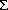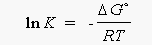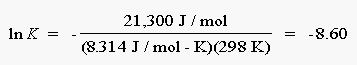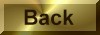Practice Problem 10

Use the following standard-state free energy of formation data to calculate the acid-dissociation equilibrium constant (Ka) at  for formic acid:

CompoundGfo(kJ/mol)

HCO2(aq)                    -372.3

H+(aq)                          0.00

HCO2-(aq)                   -351.0

Solution

We can start by writing the equation that corresponds to the acid-dissociation equilibrium for formic acid:

HCO2H(aqH+(aq) + HCO2-(aq)

We then calculate the value ofGo for this reaction:Go =Gfo(products) -Gfo(reactants)

= [1 mol H+ x 0.00 kJ/mol + 1 mol HCO2- x -351.0 kJ/mol] - [1 mol  HCO2H x -372.3 kJ/mol]

= 21.3 kJ

We now turn to the relationship betweenGo and the equilibrium constant for the reaction:Go = - RT ln K

and solve for the natural log of the equilibrium constant:Substituting the known value ofGo, R, and T into this equation gives the following result:We can now calculate the value of the equilibrium constant:

K = e-8.60 = 1.8 x10-4

The value of Ka obtained from this calculation agrees with the table value for formic acid, within experimental error.